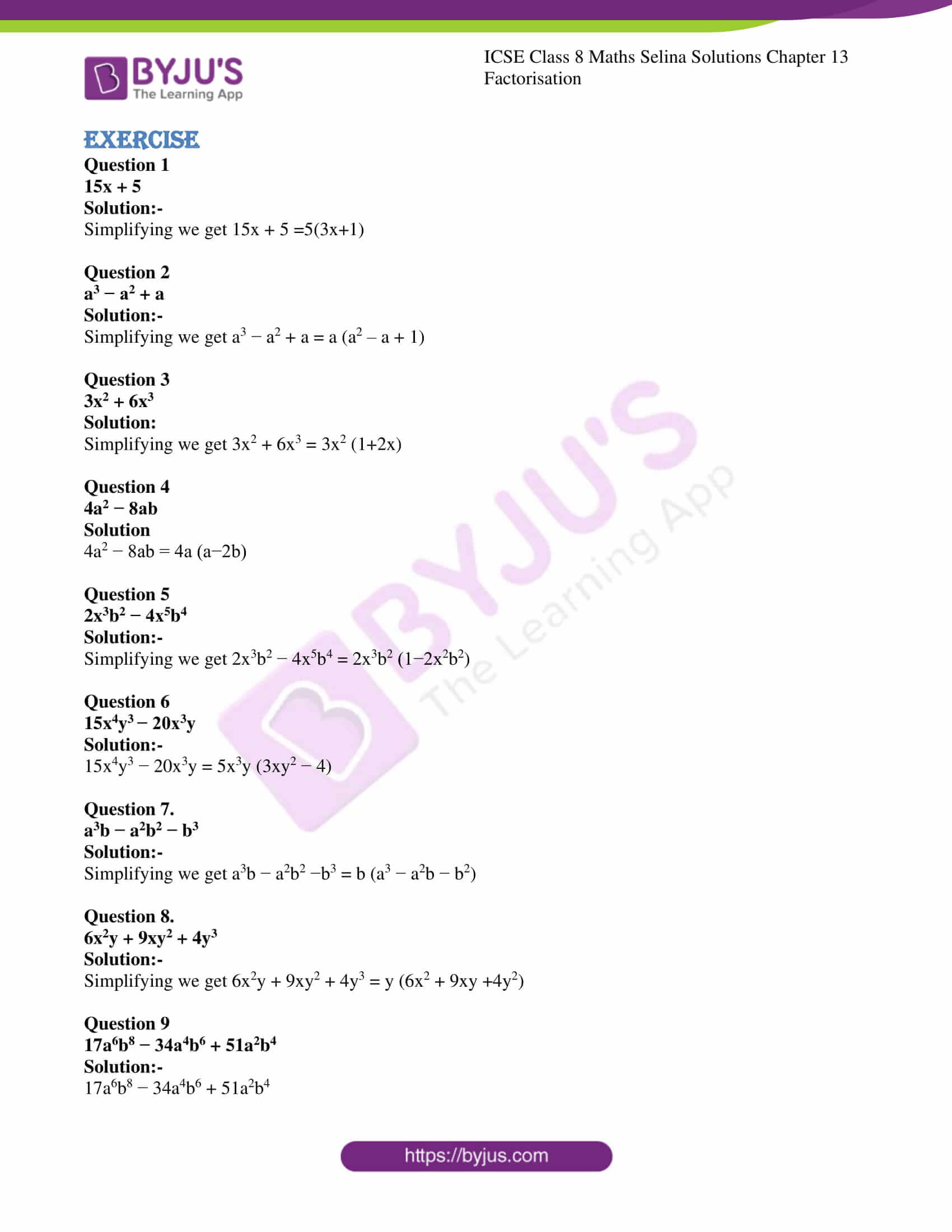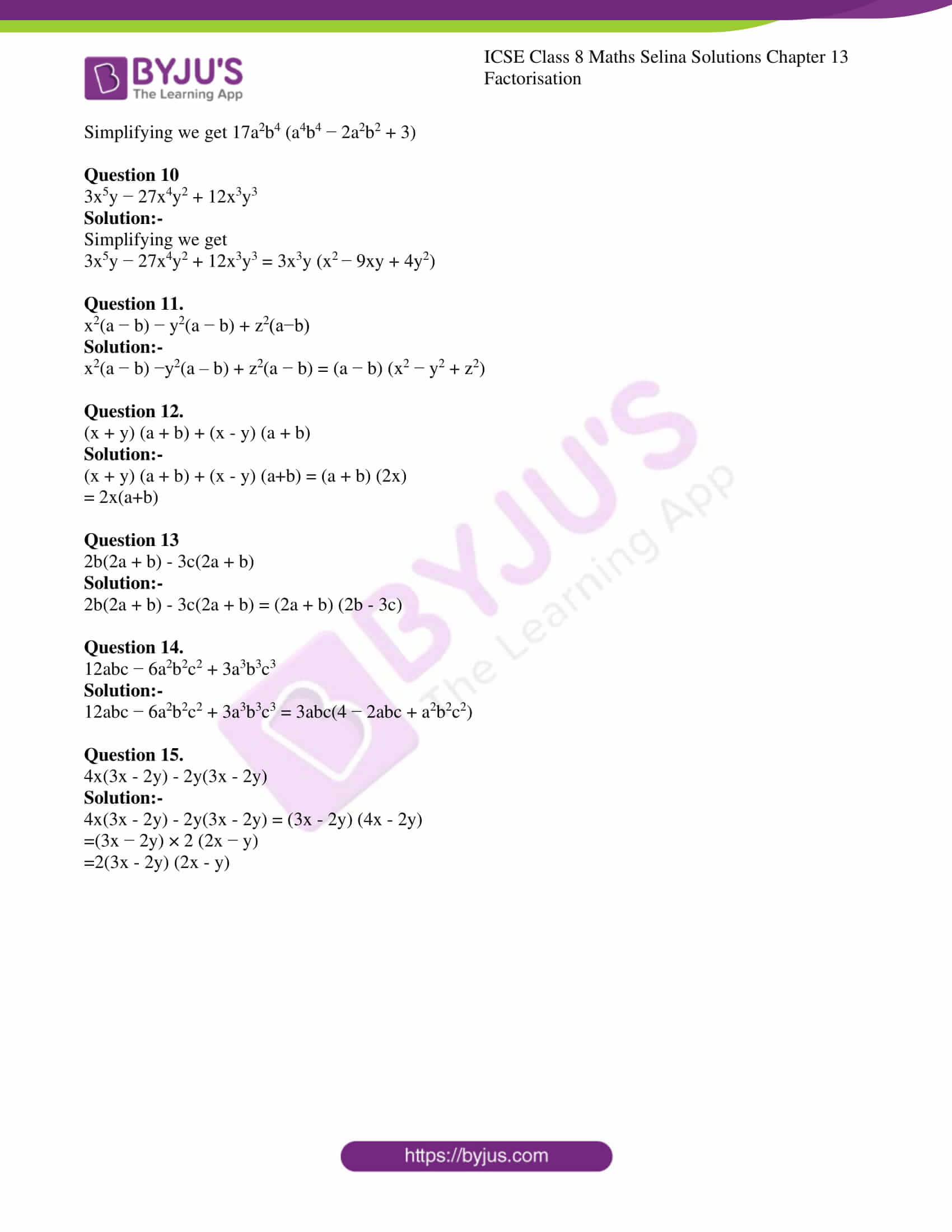# ICSE Class 8 Maths Selina Solutions Chapter 13 Factorisation

In the previous chapters, students have studied about Algebraic Expressions and Algebraic Identities. Now, in chapter 13 of class 8 maths students will learn how to express algebraic expression as a product of factors. Let’s take a simple example; the number 35 can be written as a product of 7×5. Thus, we can say that 7 and 5 are the factors of 35. Similarly, we can express the algebraic expression as a product of their factors. In this chapter, students will learn about factorization.

Once students get the concepts, they can easily solve the problems related to factorization. The questions in the exercise are simple and easy to solve. Students can expect a few questions from this chapter in the annual exam. So, solve all the problems in order to score high marks in maths paper. If students get stuck while solving the problems, they can refer to the pdf link provided below. The experts at BYJU’S have provided the answers to all the questions by solving them in the simplest way. By referring to the ICSE Class 8 Maths Selina Solutions Chapter 13 Factorisation PDF students will easily understand the method of solving these problems.ICSE Class 8 Maths Chapter 13 has only one exercise i.e 13 (A) consisting of total of 15 questions. Students can have a look at the Selina Solutions of this chapter below;

### ICSE Class 8 Maths Selina Solutions Chapter 13 Factorisation

Exercise

Question 1

15x + 5

Solution:-

Simplifying we get 15x + 5 =5(3x+1)

Question 2

a3 − a2 + a

Solution:-

Simplifying we get a3 − a2 + a = a (a2 – a + 1)

Question 3

3x2 + 6x3

Solution:

Simplifying we get 3x2 + 6x3 = 3x2 (1+2x)

Question 4

4a2 − 8ab

Solution

4a2 − 8ab = 4a (a−2b)

Question 5

2x3b2 − 4x5b4

Solution:-

Simplifying we get 2x3b2 − 4x5b4 = 2x3b2 (1−2x2b2)

Question 6

15x4y3 − 20x3y

Solution:-

15x4y3 − 20x3y = 5x3y (3xy2 − 4)

Question 7.

a3b − a2b2 − b3

Solution:-

Simplifying we get a3b − a2b2 −b3 = b (a3 − a2b − b2)

Question 8.

6x2y + 9xy2 + 4y3

Solution:-

Simplifying we get 6x2y + 9xy2 + 4y3 = y (6x2 + 9xy +4y2)

Question 9

17a6b8 − 34a4b6 + 51a2b4

Solution:-

17a6b8 − 34a4b6 + 51a2b4

Simplifying we get 17a2b4 (a4b4 − 2a2b2 + 3)

Question 10

3x5y − 27x4y2 + 12x3y3

Solution:-

Simplifying we get

3x5y − 27x4y2 + 12x3y3 = 3x3y (x2 − 9xy + 4y2)

Question 11.

x2(a − b) − y2(a − b) + z2(a−b)

Solution:-

x2(a − b) −y2(a – b) + z2(a − b) = (a − b) (x2 − y2 + z2)

Question 12.

(x + y) (a + b) + (x – y) (a + b)

Solution:-

(x + y) (a + b) + (x – y) (a+b) = (a + b) (2x)

= 2x(a+b)

Question 13

2b(2a + b) – 3c(2a + b)

Solution:-

2b(2a + b) – 3c(2a + b) = (2a + b) (2b – 3c)

Question 14.

12abc − 6a2b2c2 + 3a3b3c3

Solution:-

12abc − 6a2b2c2 + 3a3b3c3 = 3abc(4 − 2abc + a2b2c2)

Question 15.

4x(3x – 2y) – 2y(3x – 2y)

Solution:-

4x(3x – 2y) – 2y(3x – 2y) = (3x – 2y) (4x – 2y)

=(3x − 2y) × 2 (2x − y)

=2(3x – 2y) (2x – y)

To access solutions for Maths, Physics, Chemistry and Biology subjects of class 8 click on ICSE Class 8 Selina Solutions.

### Concepts Learned from ICSE Class 8 Maths Selina Solutions Chapter 13 – Factorisation

Students should solve the questions of factorization by common factor method in 3 steps as mentioned below;

1. Firstly, write each term of the expression as a product of irreducible factors.
2. Then separate the common factors.
3. Combine the remaining factors in each term in accordance with the distributive law.

To understand this method in a better way, students must look at the solved examples. After that, they will clearly get the concept. Then students can start solving the exercise problems. In case they get stuck anywhere they can refer to the solution pdf.

We hope this information on “ICSE Class 8 Maths Selina Solutions Chapter 13 Factorisation” is useful for students. Keep learning and stay tuned for further updates on ICSE and other competitive exams. To access interactive Maths and Science Videos download BYJU’S App and subscribe to YouTube Channel.